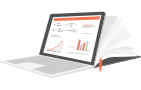# Pinball Loss FunctionBy Joannès Vermorel, February 2012

The pinball loss function, also referred to as the quantile loss, is a metric used to assess the accuracy of a quantile forecast.

Evaluating the accuracy of a quantile forecast is a subtle problem. Indeed, contrary to the classic forecasts where the goal is to have the forecast as close as possible from the observed values, the situation is biased (on purpose) when it comes to quantile forecasts. Hence the naive comparison observed vs forecasts is not satisfying. The pinball loss function returns a value that can be interpreted as the accuracy of a quantile forecasting model.

## Formula

Let $\tau$ be the target quantile, $y$ the real value and $z$ the quantile forecast, then $L_\tau$, the pinball loss function, can be written:

$$\begin{eqnarray} L_{\tau}(y,z) & = & (y - z) \tau & \textrm{ if } y \geq z \\\ & = & (z - y) (1 - \tau) & \textrm{ if } z > y \end{eqnarray}$$

The spreadsheet illustrates how to compute the pinball loss function within Microsoft Excel. The actual formula is no more complicated that most accuracy indicators such as a the MAPE.

## IllustrationThe pinball loss function (in red) has been named after its shape that looks like the trajectory of a ball on a pinball. The function is always positive and the further away from the target $y$, the larger the value of $L_\tau(y,z)$. The slope is used to reflect the desired imbalance in the quantile forecast.

## Best quantile model has lowest pinball loss

The most important result associated with the pinball loss function is that the lower the pinball loss, the more accurate the quantile forecast.

It can be proved that the function that minimizes the pinball loss delivers the optimal quantile as well. However, the formalism required for the proof goes beyond the scope of this article.

Hence, in order to compare the respective accuracy of two quantile models (say Lokad vs other), it is sufficient to compute the average pinball loss of each model over a number of time-series sufficiently large to make sure that the observed difference is statistically significant. In practice, a few hundred time-series are sufficient to assess which quantile model is the most accurate.